【GiantPandaCV导语】Vision Transformer将CV和NLP领域知识结合起来，对原始图片进行分块，展平成序列，输入进原始Transformer模型的编码器Encoder部分，最后接入一个全连接层对图片进行分类。在大型数据集上表现超过了当前SOTA模型

# 方法¶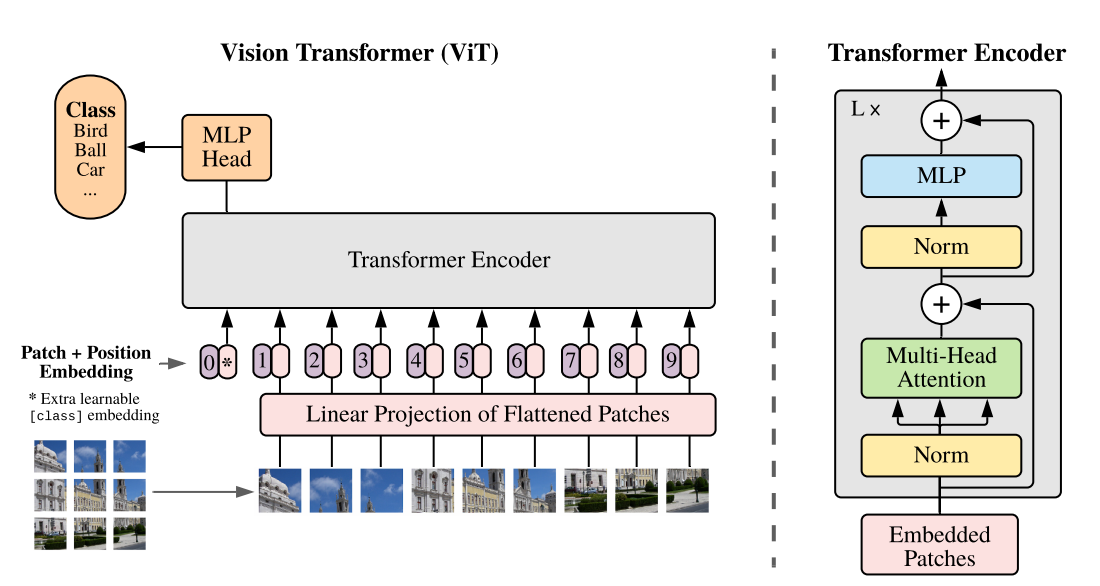### 数据处理部分¶

N = H * W /(P*P)

P*P*C

N \times (P^2·C)

x = rearrange(img, 'b c (h p1) (w p2) -> b (h w) (p1 p2 c)', p1=p, p2=p)


### Patch Embedding¶

self.patch_to_embedding = nn.Linear(patch_dim, dim)

# forward前向代码
x = rearrange(img, 'b c (h p1) (w p2) -> b (h w) (p1 p2 c)', p1=p, p2=p)
x = self.patch_to_embedding(x)


### Positional Encoding¶

self.pos_embedding = nn.Parameter(torch.randn(1, num_patches + 1, dim))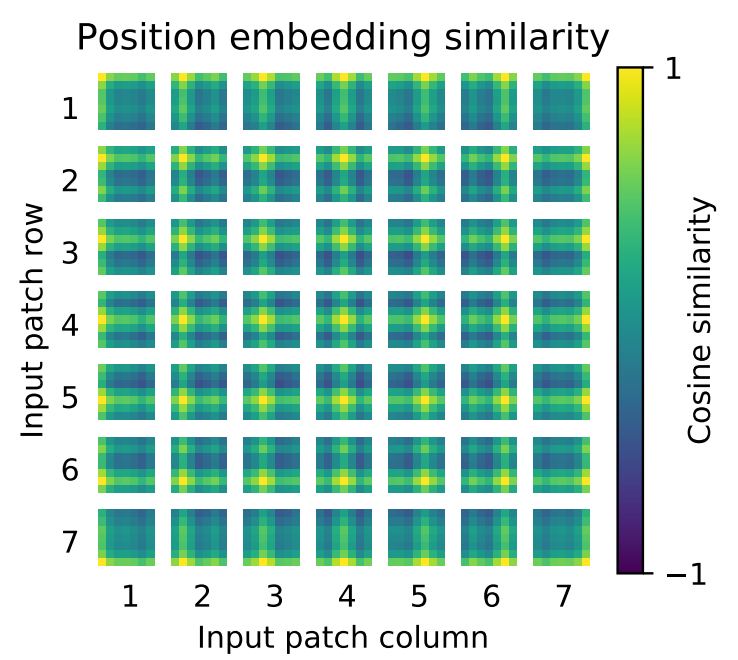### class_token¶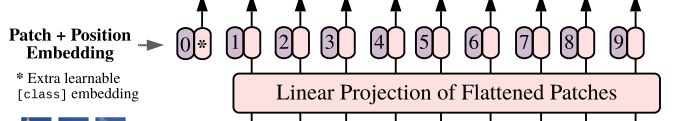# 假设dim=128，这里shape为(1, 1, 128)
self.cls_token = nn.Parameter(torch.randn(1, 1, dim))

# forward前向代码
# 假设batchsize=10，这里shape为(10, 1, 128)
cls_tokens = repeat(self.cls_token, '() n d -> b n d', b=b)
# 跟前面的分块为x（10，64， 128）的进行concat
# 得到（10， 65， 128）向量
x = torch.cat((cls_tokens, x), dim=1)


 x += self.pos_embedding[:, :(n + 1)]


### 分类¶

self.mlp_head = nn.Sequential(
nn.LayerNorm(dim),
nn.Linear(dim, mlp_dim),
nn.GELU(),
nn.Dropout(dropout),
nn.Linear(mlp_dim, num_classes)
)


self.to_cls_token = nn.Identity()
# forward前向部分
x = self.to_cls_token(x[:, 0])


class ViT(nn.Module):
def __init__(self, *, image_size, patch_size, num_classes, dim, depth, heads, mlp_dim, channels=3, dropout=0.,
emb_dropout=0.):
super().__init__()
assert image_size % patch_size == 0, 'Image dimensions must be divisible by the patch size.'
num_patches = (image_size // patch_size) ** 2
patch_dim = channels * patch_size ** 2
assert num_patches > MIN_NUM_PATCHES, f'your number of patches ({num_patches}) is way too small for attention to be effective (at least 16). Try decreasing your patch size'

self.patch_size = patch_size

self.pos_embedding = nn.Parameter(torch.randn(1, num_patches + 1, dim))
self.patch_to_embedding = nn.Linear(patch_dim, dim)
self.cls_token = nn.Parameter(torch.randn(1, 1, dim))
self.dropout = nn.Dropout(emb_dropout)

self.transformer = Transformer(dim, depth, heads, mlp_dim, dropout)

self.to_cls_token = nn.Identity()

nn.LayerNorm(dim),
nn.Linear(dim, mlp_dim),
nn.GELU(),
nn.Dropout(dropout),
nn.Linear(mlp_dim, num_classes)
)

p = self.patch_size

x = self.patch_to_embedding(x)
b, n, _ = x.shape

cls_tokens = repeat(self.cls_token, '() n d -> b n d', b=b)
x = torch.cat((cls_tokens, x), dim=1)
x += self.pos_embedding[:, :(n + 1)]
x = self.dropout(x)

x = self.to_cls_token(x[:, 0])


# 实验部分¶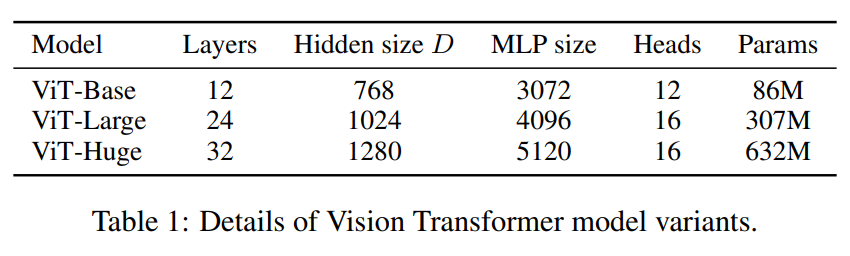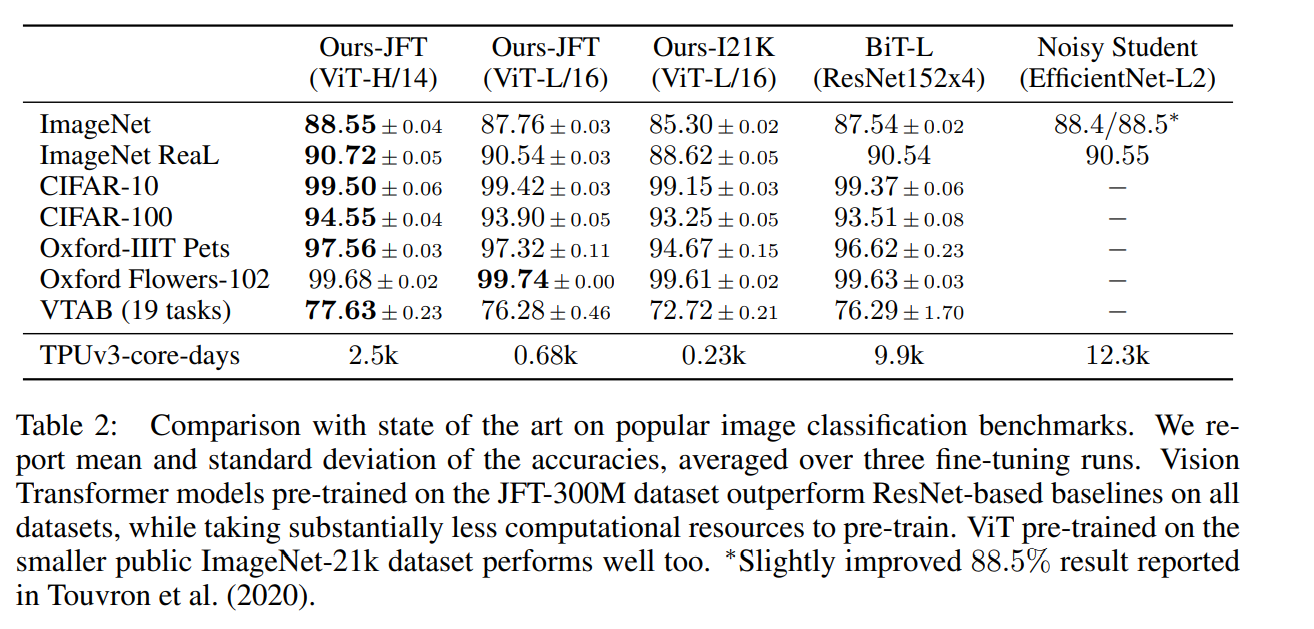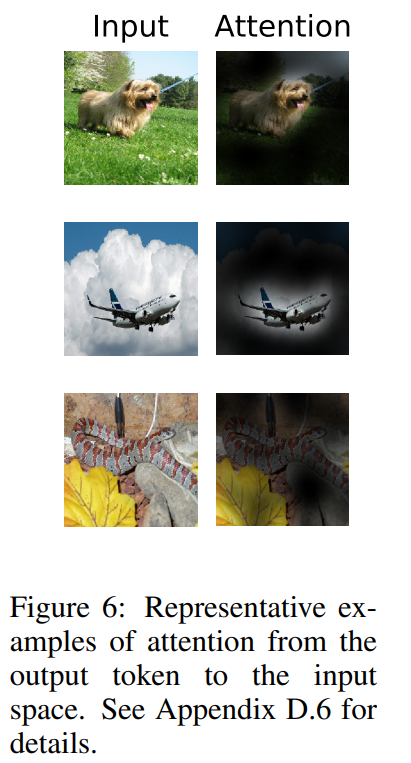# 总结¶